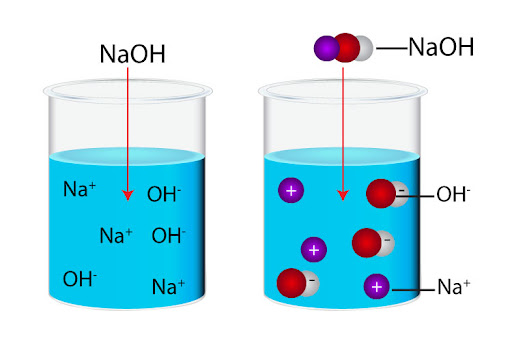# What is the pH of 0.10 mol.dm^(-3) sodium hydroxide solution, NaOH?

The pH is a determinant to acidity and basicity of its solution. The pH is calculated as a negative log of concentration of Hydrogen ions in solution.

pH= -log (H+)

We can find pH of Sodium Hydroxide solution as follows:

1. Concentration of Hydroxide Ion:

For a strong base, we expect complete dissociation of molecules so concentration is equivalent to NaOH concentration.

(OH+)= 0.1 mol/dm3

1. Concentration of Hydrogen Ion:

For any solution, the product of concentration of hydrogen ions, adn concentration of hydroxide ions is equal to ion product of water=

(H20)= (H+)(OH-)

1.00 x 10-14 = (H+)(0.1)

(H+)=1.00 x 10-13

1. Calculate the pH:

pH = - log10[1.00 x 10-13

pH=13